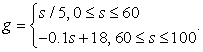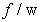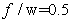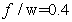﻿ Investigating Engineering Students’ Mathematical Modeling CompetencyPublications are Open
Access in this journal
Article Versions
Export Article
• Normal Style
• MLA Style
• APA Style
• Chicago Style
Research Article
Open Access Peer-reviewed

### Investigating Engineering Students’ Mathematical Modeling Competency

Chih-Hsien Huang
American Journal of Educational Research. 2018, 6(12), 1668-1672. DOI: 10.12691/education-6-12-12
Received November 05, 2018; Revised December 11, 2018; Accepted December 20, 2018

### Abstract

This study investigates university students’ modeling process in one modeling activity. The data is collected by students’ individual and group written responses to the mathematical modeling activity, video-taped group discussions and classroom observation by the researcher. The data showed that university students have difficulty in transition between different modes of mathematical representations and the classifications of variables/parameters as known or unknown, implicit or explicit, independent or dependent variables.

### 1. Introduction

Over the past ten years, it has become increasingly important to apply mathematics to other subjects, including engineering, nanotechnology, economics, and biology. Many educators and researchers in mathematics education believe that this should be reflected in the classroom via mathematical modeling activities. Students should be availed with tools in addition to school mathematics, and allowed to glimpse real-world mathematics outside the classroom. The use of models and modeling in enhancing the instruction and learning of mathematics is an indispensable means of cultivating students’ mathematical literacy, which they need in the new era of technology 1, 2, 3. Several factors, such as entrance examinations and existing teaching materials, fail to create a favorable environment for mathematical modeling in the current mathematics education situation of Taiwan. However, university students have less academic pressure, and calculus is a fundamental course in college-level mathematics and engineering education. Students need to understand the concepts of calculus and be able to apply them. For engineering students, calculus is not only a specialized subject, but also knowledge that they will need in their future workplaces. Thus, integrating modeling activities into calculus courses is a proper approach to implement mathematical modeling instruction.

This study aims to design mathematical modeling activities, based on models and modeling perspectives and embedded into calculus courses, to develop students’ mathematical modeling competency. Teaching experiments in this study used the island approach proposed by Blum and Niss 4 to integrate model-based teaching activities into formal activities for teaching calculus, and is used to avoid resistance from students who are used to traditional teaching. The ultimate purpose of the teaching experiments is to foster students’ modeling competency through a modeling process. By implementing such teaching experiments, we investigate the mathematical modeling process and competency of first year engineering students, which can be used as a reference for designing activities for teaching mathematical modeling to college students.

### 2. Theoretical Framework

Mathematical modeling instruction aims to support students in learning mathematics. Through modeling, mathematics can be used to describe, understand, and predict real-world situations. Hence, mathematical modeling can help students gain external mathematical experience, and create the connections between mathematical concepts involved in modeling activities. Mathematical modeling involves multiple processes such as mathematization, interpretation, communication, and even application. Unlike traditional problem solving, which focuses only on the representation of mathematical problems and solutions, mathematical modeling focuses on converting and interpreting contextual information, identifying potential problems, establishing models, and reinterpreting the premise, hypothesis, and possible errors of mathematical solutions. These processes are normally described in the form of stages. By following these processes, students can constantly refine and develop their mathematical models in a circular manner. Moreover, students need to be able to engage in mental activities when moving from one stage to another during the modeling process.

Competency indicates that individuals are able to make relevant decisions and implement proper actions in a real-world situation. These decisions and actions are essential for individuals in successfully handling real situations. As Blum and Leiß 6 indicated, if teaching and learning are emphasized simultaneously, an individual-oriented perspective on problem solving is necessary to better understand what students do when solving modeling problems, and to provide a better foundation for the diagnosis and involvement of educators. This study adopts the modeling cycle proposed by Galbraith and Stillman 7 as a research framework to investigate the mathematical modeling process and mathematical competency of first year engineering students.

### 3. Description of this Study

This study adopts an interpretative orientation based on anti-positivism ( 8, p. 22), regarding case studies as a research strategy for closely examining the modeling process of students. The mathematical modeling problem of this study is as follows:

A company is carrying out a cost-cutting exercise and requires your help with an investigation into how it can reduce its transport costs. The company employs a number of drivers who cover a substantial amount of mileage every day. There has recently been a large increase in their fuel costs and drivers can achieve a higher rate of miles per gallon from their vehicles by driving at a lower speed. This, however, increases journey times and the cost of the driver’s time.

The data reported in this study was gathered from three calculus classes. The entire process of each class, which lasted for 70 min, was recorded and videotaped. The subjects in this study consisted of 54 first year engineering students of a university. These students were divided into ten groups. Each group included five to six students. The researcher observed and videotaped three groups, including Groups A, D, and F. After each class, the researcher had a retrospective interview with these three groups to understand their real intentions. The researcher showed a certain group of students a video regarding their situation in class and asked them to explain their behavior in detail by asking questions, such as “How did you propose your ideas at this point?”. This article reported the modeling process of the six students in Group D. The grades of these students in calculus were approximately average. Sam, as an instructor in the teaching experiments, had 15 years experience teaching calculus in a university. He was willing to participate in this study because of his interest in fostering students’ mathematical thinking through modeling.

### 4. Major Activities of Each Class

In the first class, Sam first posed a math problem, and students had class discussions and worked on their own. After some students posed their questions, others could express their opinions. Sam guided or instructed students to implement reflection by asking students several questions such as “Why?”, “Then?”, and “How will you do it?”. Then students had group discussions and tried to understand, structure, and simplify a real-world situation and convert it into a problem statement. During this process, students gradually discovered and verified several keywords in the problem statement and were encouraged to convert a real-world problem into a problem statement based on keywords. In the second class, students needed to simply or structure a problem situation based on a problem statement, and to further generate real models. Students first held group discussions for 30 min. They were encouraged to reexamine a problem statement, and asked to create variables, parameters, constants, and symbolic representations based on keywords. In the second stage, Groups A, D, H, and G were invited to share their reports, and held class discussions. During the discussions, Sam occasionally asked student questions such as “Why did you think in this way?”, and “Do you think this is the best way?”. These questions provided students with material for discussion and enabled students to perceive the importance of examining arguments. Sam also took this opportunity to explain the similarities and differences between variables, parameters, and constants. In the third class, students first used known mathematical knowledge to solve mathematical models on their own, and then held group discussions to interpret mathematical solutions as real results.

### 5. Results

Table 1 shows the mathematical modeling competencies in each transition (cognitive activity) that were identified in this implementation of the task. Each element has two parts where key (generic) categories in the transitions between phases of the modeling cycle are indicated (in regular type), and illustrated (in capitals) with reference to the task. Evidence for selected examples of these activities is presented in the analysis of transitions that follows.

5.1. Real-world Situations → Real-world Models

In the first stage, students verified certain keywords in a problem statement, including the driving speed, costs in terms of diesel fuel and driver salary, and transportation costs. There was a mathematical observation during the inquiry process. Inquiry and mathematical observation allowed students to surpass their preconceived opinions of real-world situations, especially when students had talks with others in group discussions. For example, “The truck travels at a constant speed, ignoring traffic lights and jams” was the important concept that was simplified in group discussions.

Then, students verified the variables and limitations in the situation to investigate the key factors influencing transportation costs. For instance, John suggested that the cost of diesel is inversely proportional to transportation time. However, Mary had a different opinion, in that speed is not necessarily inversely proportional to the number of km/L of diesel a truck can get. After group discussions, relevant factors were listed as below: the factors related to driving distance, the factors related to the truck. The preceding has demonstrated that all these students could successfully generate a problem statement. Under considerations of the cost of diesel and of the driver, the students needed to derive the optimal driving speed for the truck to minimize transportation costs.

5.2. Real-world Models → Mathematical Models

In the second stage, students engaged in the work of mathematization. Students encountered the most difficulty and spent most of their time on this stage. The difficulty lied in creating mathematical properties corresponding to situational conditions and hypotheses. It seemed rather important to provide these factors; for instance, “What are parameters?” and “What are variables?”. This is also a very important process in mathematical modeling activities.

All the students in Group D had the same hypotheses on the price per L of diesel, the hourly rate of a driver, and the number of km a truck travel. However, their hypotheses on the speed of the truck and the number of km/L of diesel a truck can get were slightly different. Someone hypothesized that a truck can run 40 km at a speed of 20 km/h, and that for every 20 km/h increase in speed, the number of km/L of diesel a truck can get would be reduced by 10 km. Someone hypothesized that a truck can drive for 1 h on 1 L of diesel at a speed of 50 km/h, and that an increase in speed of 5 km/h would reduce the driving time by 0.2 h. Obviously, there was a significant difference between student performance and the meaning and purpose of mathematical modeling. Other groups of students had similar problems; thus, Sam re-explained the meaning of mathematical models, and encouraged students to hypothesize more parameters and variables.

Group D first discussed whether several keywords in the problem were parameters or variables. Gray argued that since the number of km/L of diesel a truck can get may vary with the speed of the truck, that this was a variable. Tom suggested that because the company does not often adjust salaries, the hourly rate for a truck driver can be considered as a parameter instead of a variable. Regarding the cost of diesel, in John’s opinion, even though the price of diesel may be adjusted every week, the adjustments were relatively modest. Sometimes the price rises by two cents and falls by two cents afterward, meaning that the price does not change. Therefore, the price of diesel can be hypothesized as a parameter. After a 20 min discussion, Group D reached the consensus that the heart of the question is the speed; any values directly related to the speed are variables, and the rest are parameters. Therefore, all the students finished assigning symbols to represent all the parameters and variables.

The second stage of mathematization was to construct hypotheses. A less controversial hypothesis was that a truck travels at a constant speed, ignoring traffic lights and jams. A more controversial hypothesis was the relationship between the speed of the truck and number of km/L of diesel that the truck can get, which was the most important hypothesis in this modeling activity. When working on their own, most students in Group D hypothesized that the speed of a truck is inversely proportional to the number km/L of diesel a truck can get. Such a hypothesis differed from real-world situations. Only Gray and Roberto put forward different opinions. Both of them suggested that the faster the speed, the more fuel that can be saved, based on their experiences with riding a scooter.

Hence, the students in Group D decided to investigate the data on their own regarding the speed of a truck and number of km/L of diesel a truck can get, which could serve as a basis for the subsequent discussion. These students tried to identify the relationship between the speed of a truck and number of km/L of diesel a truck can get by gathering relevant data from the Internet and dealer websites, and by calling car dealerships. The relationship between the speed of a truck and number of km/L of diesel it can get was demonstrated by the students not to be a simple linear relationship. For instance, John divided the speed of a truck into two different ranges, from 0 to 50 and from 50 to 80 km/h, and drew two segments connecting two specific points, (0,0) and (50,8), and (50,8) and (80,10), serving as the hypothesized graphical representations. After discussion, Group D hypothesized that the maximum speed was 100 km/h; beginning with 0 km/h, the number of km/L of diesel a truck can get steadily increases with increases in speed; at a speed of 60 km/h, a truck can travel 12 km on 1 L of diesel. They also hypothesized that after a truck goes over 60 km/h, the number of km/L of diesel it can get steadily decreases; at a speed of 100 km/h, a truck can travel 8 km on 1 L of diesel. Moreover, they represented their hypotheses with graphics.

After finishing its hypothesized graphical representations, Group D began with converting key factors into mathematical representations, including the traveling time, cost of a driver, and cost of diesel, and created the function of transportation costs:. At this point, Group D finished its mathematical models, including the algebraic expression of the cost function and a graphical representation of the number of km/L of diesel a truck can get.

5.3. Mathematical Models → Mathematical Solutions

In this stage, known mathematical knowledge was used to solve mathematical models. Because the form of cost functions differed from the functions with which students dealt previously, the first problem students encountered was that they did not know which variable should be differentiated. The next difficulty was how to use “g” to differentiate “s”. Students demonstrated the relationship between s and g using graphical representations; thus, they had difficulty implementing differential operations. After a 5 min discussion, they were still unable to find a solution. Therefore, Sam suggested that the students convert graphics into functional forms by using the combination of an equation of two straight lines. After about 5 min, Group D represented the relationship between s and g with an algebraic expression:Finally, they used the concept of first-order derivatives to determine a mathematical solution. However, students wondered why the solution for the optimal speed contained parameters, because they thought that the form of an answer should be a number, based on their previous learning experiences. Sam discovered that most students had questions about the form of the mathematical solution. Hence, in class discussions, he explained the purpose and meaning of mathematical modeling and the meaning of a mathematical solution that included parameters.

5.4. Mathematical Solutions → Real-world Meaning of Solutions

In this stage, students interpreted mathematical solutions as real results. In the last stage, Group D discovered that the optimal speed was correlated with the value of. They also understood that they needed to propose possible results based on the value of. Tom suggested that when, the optimal speed and lowest transportation costs can be obtained. However, he did not consider the hourly rate of the driver to be unreasonable, which was only approximately 60 NTD when. There were three students suggested thatshould be 0.4 when the price per liter of diesel was 29 or 30 NTD. There were two students listed several solutions such as, s=74.6; when, s=65.9; and when, when, s=55.6. They did not provide a single solution; instead, provided clients with the suggestion that the value ofbe between 0.3 and 0.4.

### 6. Conclusions

This study emphasizes student reflection on mathematical understanding, mathematization and analysis of mathematical systems, and the interpretation of results to a given real-world problem, providing students with an opportunity to learn mathematics in a different way. This study replaced extrema problems in calculus courses with the mathematical modeling activities of reducing transportation costs. Through mathematical modeling instruction, students can gradually develop their mathematical modeling competency by working on their own and through discussion with their peers. The analysis results of research data show that a fundamental and important problem encountered by students is their failure to recognize variables and parameters; and whether these values are known or unknown, obscure or clear, or independent or related. Without this fundamental knowledge, students may have difficulty engaging in mathematical modeling activities, especially during the process of mathematization. Therefore, the insufficient ability of students to categorize variables and parameters should not be ignored. Educators should help students in establishing useful relationships required by mathematical problems. Another obvious problem is representational change. Representational tools and systems such as tables, graphics, and drawings are important parts of the mathematical modeling process. This research result accords with the argument emphasized by 3: that representational fluency plays an important role in the model-documentation principle of mathematical modeling.

The study results verify the cognitive activities in which students engaged and the competency required during the mathematical modeling process of solving the problem of reducing transportation costs. Researchers and teachers can use the transitional framework proposed in this study to verify whether students have the specific abilities necessary to successfully finish particular mathematical modeling problems. Teachers who want to implement mathematical modeling instruction can also use the transitional framework to ensure that students are able to develop their mathematical modeling competency, even though not every modeling activity includes every element. From the perspective of student learning, the transitional framework can be used to predict difficulties that students may encounter.

### References

  Burkhardt, H., “Modelling in Mathematics Classrooms: reflections on past developments and the future”, Zentralblatt für Didaktik der Mathematik, 38 (2), 178-195, 2006. In article View Article  English, C., “Modelling for the New Millennium”, In C. Hoyles, C. Morgan & G. Woodhouse (Eds.), Rethinking the mathematics curriculum (pp. 118-129). London: Falmer Press, 1999. In article  Lesh. R. and Doerr H. M., Beyond constructivism: models and modeling perspectives on mathematics problem solving, learning, and teaching,. American, Lawrence Erlbaum Associates Inc, 2003. In article  Blum, W. and Niss, M., “Applied mathematical problem solving, modelling, applications, and links to other subjects - state, trends and issues in mathematics instruction”, Educational Studies in Mathematics, 22, 37-68, 1991. In article View Article  Maaß, K., “What are modelling competencies?”, Zentralblatt für Didaktik der Mathematik, 38(2), 113-142, 2006. In article View Article  Blum, W. and Leiß, D., “How do students and teachers deal with mathematical modeling problems? The example “Sugarloaf” and the DISUM project”, In C. Haines, P. Galbraith, W. Blum & S. Khan (Eds.), Mathematical modelling (ICTMA12): Education, engineering and economics (pp. 222-231). Chichester, UK: Horwood, 2006. In article  Galbraith, P. and Stillman, G., “A framework for identifying student blockages during transitions in the modelling process”, Zentralblatt für Didaktik der Mathematik, 38(2), 143-162, 2006. In article View Article  Cohen, L., Manion, L., and Morrison, K., Research Methods in Education, (5th edition). London: Routledge, 2000. In articleThis work is licensed under a Creative Commons Attribution 4.0 International License. To view a copy of this license, visit http://creativecommons.org/licenses/by/4.0/